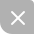# Swordsman —— 输入模板

Problem Description
Lawson is a magic swordsman with k kinds of magic attributes v1,v2,v3,…,vk. Now Lawson is faced with n monsters and the i-th monster also has k kinds of defensive attributes ai,1,ai,2,ai,3,…,ai,k. If v1≥ai,1 and v2≥ai,2 and v3≥ai,3 and … and vk≥ai,k, Lawson can kill the i-th monster (each monster can be killed for at most one time) and get EXP from the battle, which means vj will increase bi,j for j=1,2,3,…,k.
Now we want to know how many monsters Lawson can kill at most and how much Lawson’s magic attributes can be maximized.

Input
There are multiple test cases. The first line of input contains an integer T, indicating the number of test cases. For each test case:
The first line has two integers n and k (1≤n≤105,1≤k≤5).
The second line has k non-negative integers (initial magic attributes) v1,v2,v3,…,vk.
For the next n lines, the i-th line contains 2k non-negative integers ai,1,ai,2,ai,3,…,ai,k,bi,1,bi,2,bi,3,…,bi,k.
It’s guaranteed that all input integers are no more than 109 and vj+∑i=1nbi,j≤109 for j=1,2,3,…,k.

It is guaranteed that the sum of all n ≤5×105.
The input data is very large so fast IO (like fread) is recommended.

Output
For each test case:
The first line has one integer which means the maximum number of monsters that can be killed by Lawson.
The second line has k integers v′1,v′2,v′3,…,v′k and the i-th integer means maximum of the i-th magic attibute.

Sample Input
1
4 3
7 1 1
5 5 2 6 3 1
24 1 1 1 2 1
0 4 1 5 1 1
6 0 1 5 3 1

Sample Output
3
23 8 4

Hint

For the sample, initial V = [7, 1, 1]
① kill monster #4 (6, 0, 1), V + [5, 3, 1] = [12, 4, 2]
② kill monster #3 (0, 4, 1), V + [5, 1, 1] = [17, 5, 3]
③ kill monster #1 (5, 5, 2), V + [6, 3, 1] = [23, 8, 4]
After three battles, Lawson are still not able to kill monster #2 (24, 1, 1)
because 23 < 24.

#include<bits/stdc++.h>
using namespace std;

namespace fastIO {
#define BUF_SIZE 100000
bool IOerror = 0;
inline char nc() {
static char buf[BUF_SIZE], *p1 = buf + BUF_SIZE, *pend = buf + BUF_SIZE;
if(p1 == pend) {
p1 = buf;
pend = buf + fread(buf, 1, BUF_SIZE, stdin);
if(pend == p1) {
IOerror = 1;
return -1;
}
}
return *p1++;
}
inline bool blank(char ch) {
return ch == ' ' || ch == '\n' || ch == '\r' || ch == '\t';
}
char ch;
while(blank(ch = nc()));
if(IOerror) return;
for(x = ch - '0'; (ch = nc()) >= '0' && ch <= '9'; x = x * 10 + ch - '0');
}
#undef BUF_SIZE
};
using namespace fastIO;

#define pa pair<int,int>
#define mp(a,b) make_pair(a,b)
priority_queue<pa,vector<pa>, greater<pa> >Q;
int main()
{
int t;
scanf("%d",&t);
while(t--)
{
int n,k;
int s;
priority_queue<pa,vector<pa>, greater<pa> >Q;
for(int i=1;i<=k;i++)
for(int i=1;i<=n;i++)
{
for(int j=1;j<=k;j++)
Q.push({def[i],i});
for(int j=1;j<=k;j++)
}
int ans=0;
while(1)
{
int lastans=ans;
for(int i=1;i<k;i++)
{
while(!Q[i].empty()&&Q[i].top().first<=s[i])
{
int pos=Q[i].top().second;
Q[i].pop();
Q[i+1].push({def[pos][i+1],pos});

}
}
while(!Q[k].empty()&&Q[k].top().first<=s[k])
{
int pos=Q[k].top().second;
Q[k].pop();
ans++;
for(int i=1;i<=k;i++)
}
if(ans==lastans)
break;
}
printf("%d\n",ans);
for(int i=1;i<=k;i++)
printf("%d%c",s[i],i==k?'\n':' ');
}
return 0;
}UP更新不错过~
08-14326
08-14133
08-16263
08-15269
08-14178
08-14200
08-15154
08-14235
08-17165
08-13145
01-239527
09-1855
09-0499
08-14186
08-13182
08-1498
08-14129

### “相关推荐”对你有帮助么？

•非常没帮助
•没帮助
•一般
•有帮助
•非常有帮助被折叠的  条评论 为什么被折叠?到【灌水乐园】发言¥2 ¥4 ¥6 ¥10 ¥20余额支付 (余额：-- )扫码支付获取中扫码支付点击重新获取扫码支付1.余额是钱包充值的虚拟货币，按照1:1的比例进行支付金额的抵扣。
2.余额无法直接购买下载，可以购买VIP、C币套餐、付费专栏及课程。余额充值